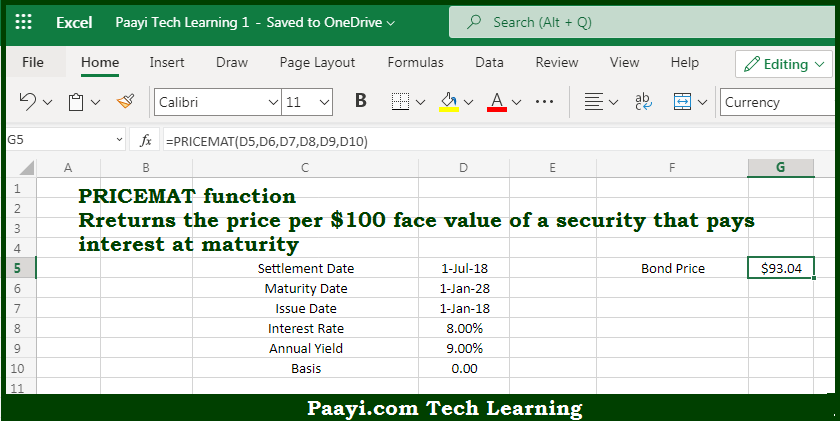# Learn How to Use Microsoft Excel PRICEMAT Function

Written by | 0 Comments | 483 Views

In this article, you will learn how to use the Microsoft Excel PRICEMAT function and its prime function in Microsoft Excel. You will also get to know the Microsoft Excel PRICEMAT function return value and syntax with the help of some examples.

Microsoft Excel PRICEMAT Function

The main purpose of the Microsoft Excel PRICEMAT function is to get the price per \$100 interest at maturity. That implies, with the help of the PRICEMAT function you can able to return the price per \$100 face value of a security that pays interest at maturity. So, with the help of the PRICEMAT function, you can able to get the price per \$100 interest at maturity.

Return Value of PRICEMAT Function

The return value will be the bond price.

Syntax of PRICEMAT Function

=PRICEMAT(sd, md, is, rate, yld, [basis])

Where the arguments:

• sd: This is the settlement date of the security.
• md: This is the maturity date of the security.
• id: This is the issue date of the security.
• rate: This is the interest rate of the security on issuing date.
• yld: This is the annual yield of the security.
• basis: This is the day count basis, and defaults to 0 (optional).

### How to Use Microsoft Excel PRICEMAT Function?So we know that Microsoft Excel PRICEMAT function you can able to get the price per \$100 interest at maturity. That implies, with the help of the PRICEMAT function you can able to return the price per \$100 face value of a security that pays interest at maturity. So, with the help of the PRICEMAT function, you can able to get the price per \$100 interest at maturity.